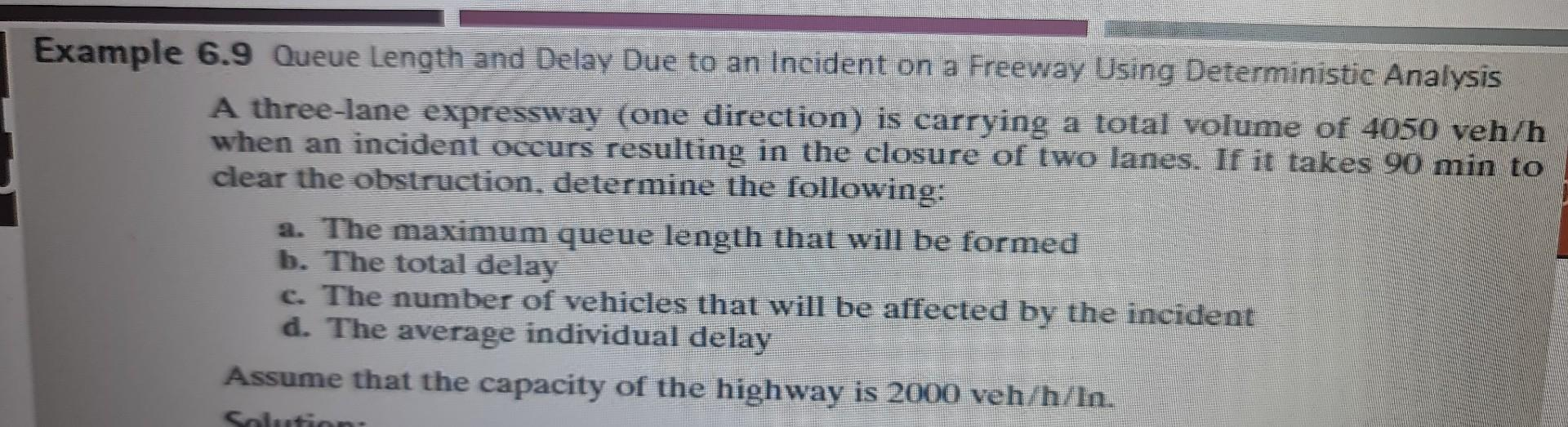Home / Expert Answers / Civil Engineering / nbsp-6-9-queue-length-and-delay-due-to-an-incident-on-a-freeway-using-deterministic-analysis-a-pa416

# (Solved):   6.9 Queue Length and Delay Due to an Incident on a Freeway Using Deterministic Analysis A ...6.9 Queue Length and Delay Due to an Incident on a Freeway Using Deterministic Analysis A three-lane expressway (one direction) is carrying a total volume of $$4050 \mathrm{veh} / \mathrm{h}$$ when an incident occurs resulting in the closure of two lanes. If it takes $$90 \mathrm{~min}$$ to clear the obstruction, determine the following: a. The maximum queue length that will be formed b. The total delay c. The number of vehicles that will be affected by the incident d. The average individual delay Assume that the capacity of the highway is $$2000 \mathrm{veh} / \mathrm{h} / \mathrm{hn}$$.

We have an Answer from Expert